LEVEL 6:

19.    Coal is a non-renewable energy resource.

(a)      Give two other non-renewable energy resources.

1

2.

2 marks

Wood is a renewable energy resource.

(b)      Why can wood be described as a renewable energy resource?

1 mark

(c)      Give two other renewable energy resources.

1.      ..

2..

2 marks

(d)      Complete the statement below to describe what happens when wood burns.

When wood burns, chemical energy in the wood is transformed

into energy, which is transferred to the surroundings.

1 mark

20.     A bookshelf is 1 metre above the floor.  As a book falls from the shelf to the floor it loses 7.5 J of potential energy.

(a)      (i)       Tick the box by the correct statement about the kinetic energy of the book.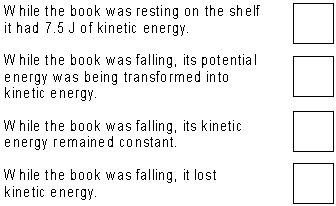1 mark

How much kinetic energy does the book have just before it hits the floor?   J

1 mark

(b)      When the book hits the floor it stops and loses all its kinetic energy.

What happens to this energy?

2 marks

21.     Energy comes from a variety of sources.

Complete the table below.

The first one has been done for you.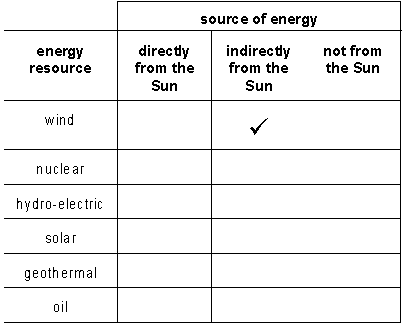5 marks

22.     In July 1994, fragments of the comet shoemaker-Levy 9 were pulled into the planet Jupiter, causing a series of enormous collisions.

(a)      The fragments of comet were pulled towards Jupiter by gravity.  How did the gravitational force vary with the mass of the fragment?

1 mark

(b)      As each fragment approached Jupiter, the gravitational force on it changed. Describe the change.

...                               1 mark

(c)      Tick the correct box to complete the statement.

Between the different fragments of the comet

there was an attractive force           there was a repulsive force          there was no force

1 mark

The comet was in fragments because, in July 1992, it had passed very close to Jupiter and had broken up.

(d)      Tick the correct box to describe the gravitational force per kilogram on different sides of the comet as it passed close to Jupiter.

all parts of the comet were pulled towards Jupiter equally strongly

all parts of the comet were pulled towards Jupiter,
but the side facing towards the planet was pulled more strongly

all parts of the comet were pulled towards Jupiter, but the side
facing away from the planet was pulled more strongly

the side of the comet facing towards Jupiter was pulled towards the planet but the side facing away was repelled

1 mark

(e)      Tick the correct box to show what gravitational force (if any) the comet exerted on Jupiter.

no force                            an attractive force               a repulsive force

1 mark

23.     The diagram shows an electromagnet used in a door lock.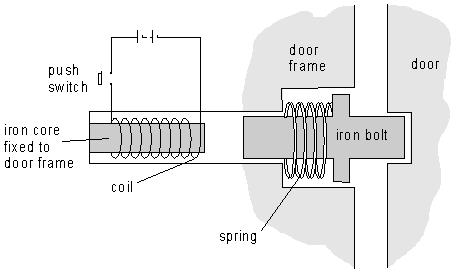(a)      The push switch is closed and the door unlocks.  Explain in detail how this happens.

3 marks

(b)      The switch is released and the door locks.  Explain in detail how this happens.

2 marks

24.     The diagram shows a cyclist at different positions as he cycles over a humpback bridge.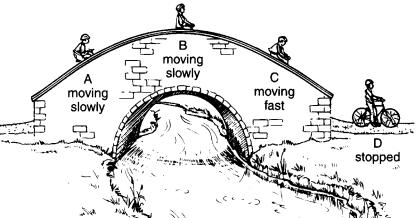(a)      (i)       Where does the cyclist have the most kinetic energy?
Tick the correct box.

 A B C D

1 mark

(ii)      Where does the cyclist have the most potential energy?
Tick the correct box.

 A B C D

1 mark

(iii)     Where does the cyclist have the least potential energy?
Tick the correct box.

 A B C D

1 mark

(b)      The cyclist used some energy to cycle over the bridge.  Where was this energy transferred to by the time the cyclist reached position D?

1 mark

25.     An experiment is set up as shown.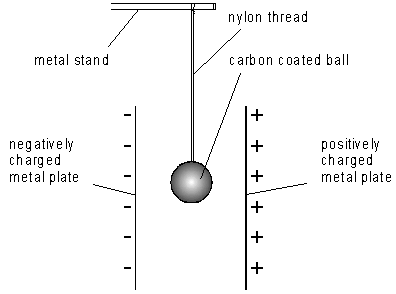The plates are charged. The ball is moved across to touch the positive plate, and becomes positively charged. It is repelled from the plate.

(a)      (i)       Why is the ball repelled from the positive plate?

1 mark

(ii)      Why is the ball pulled towards the negative plate?

1 mark

(b)      The ball reaches the negatively charged plate, touches it, and moves back quickly. Explain why it moves away quickly.

2 marks

(c)      Nylon is an insulator. Explain why an insulator is used to hold up the ball rather than a conductor like copper.

1 mark

26.     The diagram represents a conductor.  It has equal numbers of positive and
negative charges.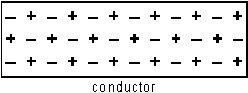(a)      In the diagram, the + signs and the – signs are shown throughout the conductor.  This represents the pattern of positive and negative charges in a real conductor. Why are the charges spread out instead of forming areas of positive charge and areas of negative charge?

1 mark

A negatively charged object is brought near to the conductor.  This changes the position of charges in the conductor.

(b)      (i)       On the diagram below, draw the new pattern of positive and negative charges in the conductor.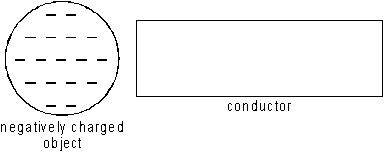2 marks

(ii)      Why does the pattern of positive and negative charges change in this way?

1 mark

(c)      One end of the conductor is attracted to the negativity charged object and the other end is repelled.

The force of attraction is larger than the force of repulsion.  Explain why.

1 mark

27.     The drawing shows what happens to most of the energy in the food that a hen eats in one day.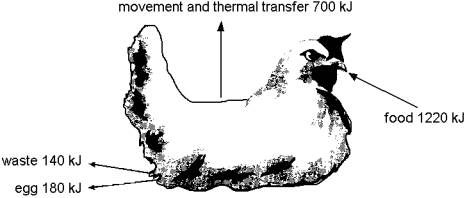(a)      In the cells of the hen's body, energy is released from food by respiration
Complete the word equation for this process.

Glucose +  ® +

1 mark

(b)      (i)       Calculate the total energy which remains in the body of the hen

kJ                                    1 mark

(ii)      What is this energy used for?

1 mark

(c)      Suggest how farmers might reduce the amount of energy which hens lose each day by thermal transfer.

1 mark

(d)      Farmers can reduce the amount of energy which is transferred by movement and thermal transfer from hens. Suggest two reasons why this is costeffective.

1.

2.

2 marks